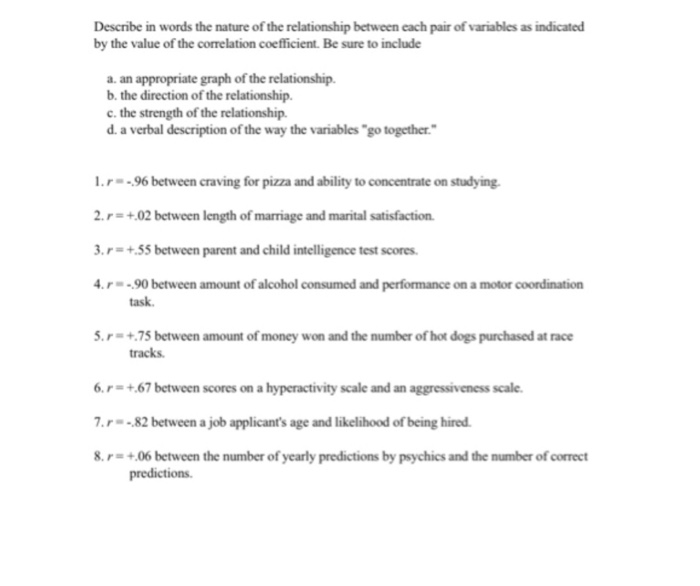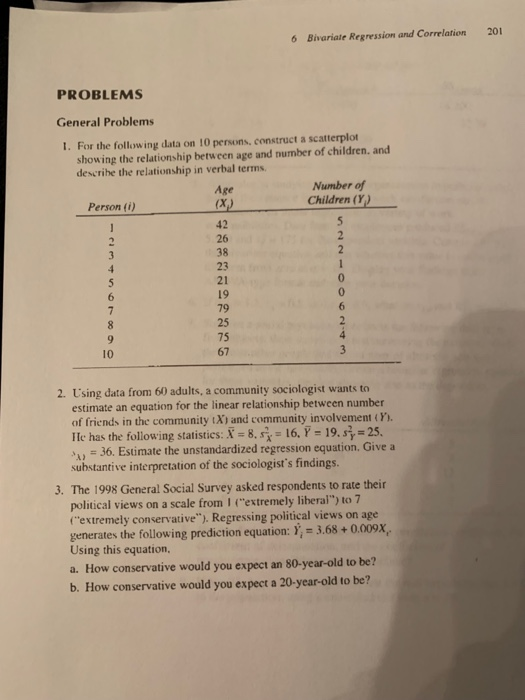Homework Help Question & Answers

# Describe in words the nature of the relationship between each pair of variables as indicated by the value of the correlation coefficient

Describe in words the nature of the relationship between each pair of variables as indicated by the value of the correlation coefficient. Be sure to include

a. the direction of the relationship.
b. the strength of the relationship.
c. a verbal description of the way the variables "go together."
d. an appropriate graph of the relationship. (for the graphing portion, describe what the scatterplot should look like in terms of slope, linear characteristics, etc)

1. r = -.96 between craving for pizza and ability to concentrate on studying.
2. r = +.02 between length of marriage and marital satisfaction.
3. r = +.55 between parent and child intelligence test scores.
4. r = -.90 between amount of alcohol consumed and performance on a motor coordination task.
5. r = +.75 between amount of money won and the number of hot dogs purchased at race tracks.
6. r = +.67 between scores on a hyperactivity scale and an aggressiveness scale.
7. r = -.82 between a job applicant's age and likelihood of being hired.
8. r = +.06 between the number of yearly predictions by psychics and the number of correct predictions.

I NEED HELP AM NOT UNDERSTANDING THIS.

#### Homework Answers

Answer #1
Positive r, both variables increase/decrease together. The direction is from lower left to upper right.

Negative r, one variable increases while the other decreases. Direction is from upper left to lower right.

r = ± 1 = prefect predictability, straight line. r = 0 = no predictability, scores spread randomly. As approach 1, the spread gets less and less, and predictability gets greater.

The above information should help you answer the above questions.
answered by: duuu
Know the answer?
Your Answer:

#### Post as a guest

Your Name:

What's your source?

#### Earn Coin

Coins can be redeemed for fabulous gifts.

Not the answer you're looking for? Ask your own homework help question. Our experts will answer your question WITHIN MINUTES for Free.
Similar Homework Help Questions
• ### Describe in words the nature of the relationship between each pair of variables as indicated by...Describe in words the nature of the relationship between each pair of variables as indicated by the value of the corelation coefficient. Be sure to include a. an appropriate graph of the relationship. b. the direction of the relationship c. the strength of the relationship d. a verbal description of the way the variables "go together I.r--96 between craving for pizza and ability to concentrate on studying. 2.r- +02 between length of marriage and marital satisfaction .r-+55 between parent and...

• ### 201 Bivariate Regression and Correlation 6 PROBLEMS General Problems 1. For the following data on...I need questions 1-3 & 6-10 answered with shown calculated work. 201 Bivariate Regression and Correlation 6 PROBLEMS General Problems 1. For the following data on 10 persons, construct a scatterplot showing the relationship between age and number of children. and describe the relationship in verbal terms Number o Children (Y) Age Person (i) 42 26 38 23 21 19 79 25 75 67 9 10 2. Using data from 60 adults, a community sociologist wants to estimate an equation...

• ### A Final Report SPSS

All the information needed is below. Because the final project is done in stagesit ONLY goes up through Week 6…Please note that.Journal ArticlesHowell, D. C., Huessy, H. R., &Hassuk, B. (1985). Fifteen-year follow-up of a behavioral history of attention deficit disorder. Pediatrics, 76(2), 185–190.Retrieved January 27, 2006, from Academic Search Premier database. Accession Number (AN) 4733982.Final ProjectThe project is to be completed in stages. I have listed the first 6 stages that need to be completed. Starting in the first...

Free Homework App

Scan Your Homework
to Get Instant Free Answers
Need Online Homework Help?

Get Answers For Free
Most questions answered within 3 hours.